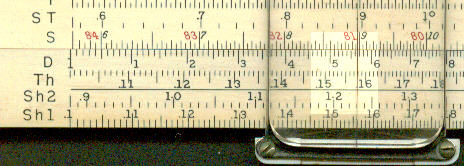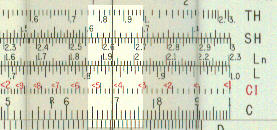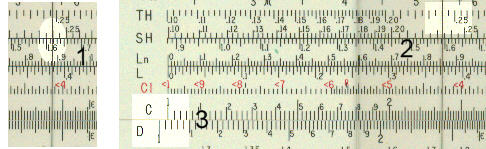Hyperbolic functions

Hyperbolic functions are found useful in two main areas:

• electrical engineering - this is their principal use.

• they define the shape of a catenary curve (when a rope is suspended between points).

The three main hyperbolic functions are:

 sinh = (ex - e-x) /2 The hyperbolic sine of x, pronounced "shine" cosh =  (ex + e-x) /2 The hyperbolic cosine of x, pronounced "cosh" tanh = (ex - e-x) / (ex + e-x) The hyperbolic tangent of x, pronounced "tansh"

The Keuffel and Esser N4083-3 Log-log Duplex Vector has two sinh scales, marked Sh1 and Sh2, and one tanh scale, marked, Th.

The sinh scales cover the ranges of x from 0.1 to 1 and 1 to 10.  For x<0.1, sinh x = x so no scale for smaller values is needed. The tanh scale covers the range 0.1 to 3. For x < 0.1, tanh x = x and for x>3, tanh = 1, so no other scales are needed. The scales are arranged so that x is on the Sh or Th scale and the value of the function is on the D scale.

The example below shows the value of x as 1.23 on the Sh2 scale and the value sinh x as 1.565 on the D scale.On the Pickett N 4P-T, the hyperbolic functions are on the slide, not the stock. The rule covers the same range of values of x and also has two sinh and 1 tanh scale. The only difference is that the values of the functions are read on the C scale and not the D scale.

The following shows x as 0.85 on the Th scale and tanh x as 0.69 on the C scale.No separate scales are provide for cosh since cosh x = sinh x / tanh x.

On the Pickett this is quite easy. For example to calculate cosh 0.24. Align the scales so that the C and D scales are coincident and set the cursor line to 0.24 on the sinh scale ( number 1, first figure below).  Next move the slide so that 0.24 on the tanh scale is under the cursor line (number 2, second figure below). Finally read the answer, 1.03, on the D scale against 1.0 on the C scale.On the K & E this calculation is not quite so easy as it involves use of the C scale on the opposite face of the rule but similar principles apply.

On some rules without hyperbolic scales, the Aristo 0968 for example, the LLn (i.e. ex ) scales and LL0n (i.e. e-x ) are arranged so that both values can be read at the same time from which the hyperbolic functions can be calculated with appropriate formula.